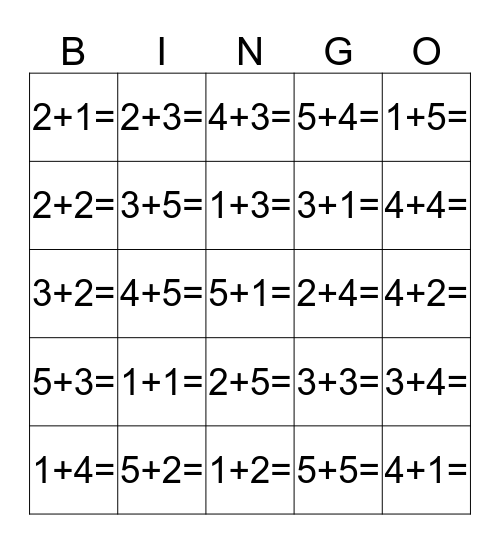# Site WordsThis bingo card has 25 words: 1+1=, 1+2=, 1+3=, 1+4=, 1+5=, 2+1=, 2+2=, 2+3=, 2+4=, 2+5=, 3+1=, 3+2=, 3+3=, 3+4=, 3+5=, 4+1=, 4+2=, 4+3=, 4+4=, 4+5=, 5+1=, 5+2=, 5+3=, 5+4= and 5+5=.

## Play Online